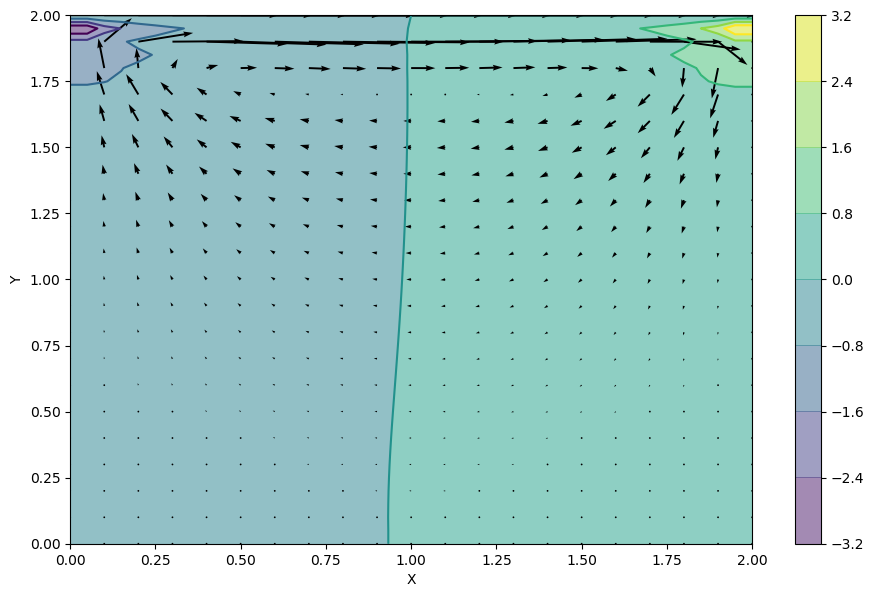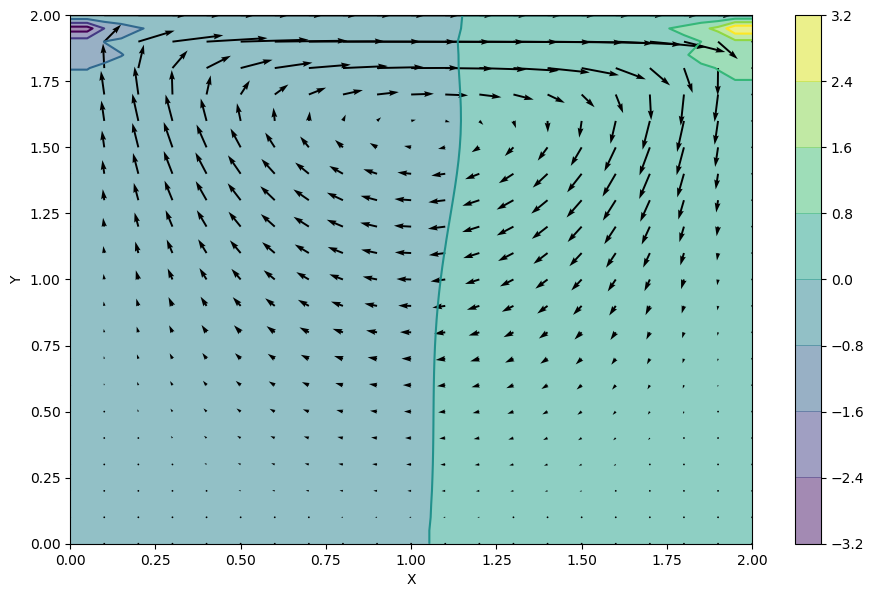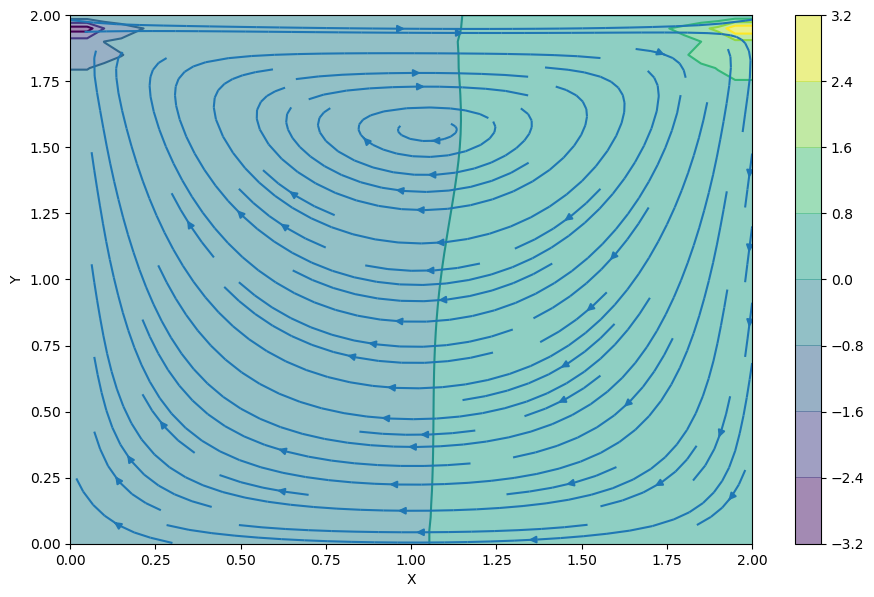Text provided under a Creative Commons Attribution license, CC-BY. All code is made available under the FSF-approved BSD-3 license. (c) Lorena A. Barba, Gilbert F. Forsyth 2017. Thanks to NSF for support via CAREER award #1149784.

# 12 steps to Navier–Stokes¶

The final two steps in this interactive module teaching beginning CFD with Python will both solve the Navier–Stokes equations in two dimensions, but with different boundary conditions.

The momentum equation in vector form for a velocity field $\vec{v}$ is:

$$\frac{\partial \vec{v}}{\partial t}+(\vec{v}\cdot\nabla)\vec{v}=-\frac{1}{\rho}\nabla p + \nu \nabla^2\vec{v}$$

This represents three scalar equations, one for each velocity component $(u,v,w)$. But we will solve it in two dimensions, so there will be two scalar equations.

Remember the continuity equation? This is where the Poisson equation for pressure comes in!

## Step 11: Cavity Flow with Navier–Stokes¶

Here is the system of differential equations: two equations for the velocity components $u,v$ and one equation for pressure:

$$\frac{\partial u}{\partial t}+u\frac{\partial u}{\partial x}+v\frac{\partial u}{\partial y} = -\frac{1}{\rho}\frac{\partial p}{\partial x}+\nu \left(\frac{\partial^2 u}{\partial x^2}+\frac{\partial^2 u}{\partial y^2} \right)$$$$\frac{\partial v}{\partial t}+u\frac{\partial v}{\partial x}+v\frac{\partial v}{\partial y} = -\frac{1}{\rho}\frac{\partial p}{\partial y}+\nu\left(\frac{\partial^2 v}{\partial x^2}+\frac{\partial^2 v}{\partial y^2}\right)$$$$\frac{\partial^2 p}{\partial x^2}+\frac{\partial^2 p}{\partial y^2} = -\rho\left(\frac{\partial u}{\partial x}\frac{\partial u}{\partial x}+2\frac{\partial u}{\partial y}\frac{\partial v}{\partial x}+\frac{\partial v}{\partial y}\frac{\partial v}{\partial y} \right)$$

From the previous steps, we already know how to discretize all these terms. Only the last equation is a little unfamiliar. But with a little patience, it will not be hard!

### Discretized equations¶

First, let's discretize the $u$-momentum equation, as follows:

$$\begin{split} & \frac{u_{i,j}^{n+1}-u_{i,j}^{n}}{\Delta t}+u_{i,j}^{n}\frac{u_{i,j}^{n}-u_{i-1,j}^{n}}{\Delta x}+v_{i,j}^{n}\frac{u_{i,j}^{n}-u_{i,j-1}^{n}}{\Delta y} = \\ & \qquad -\frac{1}{\rho}\frac{p_{i+1,j}^{n}-p_{i-1,j}^{n}}{2\Delta x}+\nu\left(\frac{u_{i+1,j}^{n}-2u_{i,j}^{n}+u_{i-1,j}^{n}}{\Delta x^2}+\frac{u_{i,j+1}^{n}-2u_{i,j}^{n}+u_{i,j-1}^{n}}{\Delta y^2}\right) \end{split}$$

Similarly for the $v$-momentum equation:

$$\begin{split} &\frac{v_{i,j}^{n+1}-v_{i,j}^{n}}{\Delta t}+u_{i,j}^{n}\frac{v_{i,j}^{n}-v_{i-1,j}^{n}}{\Delta x}+v_{i,j}^{n}\frac{v_{i,j}^{n}-v_{i,j-1}^{n}}{\Delta y} = \\ & \qquad -\frac{1}{\rho}\frac{p_{i,j+1}^{n}-p_{i,j-1}^{n}}{2\Delta y} +\nu\left(\frac{v_{i+1,j}^{n}-2v_{i,j}^{n}+v_{i-1,j}^{n}}{\Delta x^2}+\frac{v_{i,j+1}^{n}-2v_{i,j}^{n}+v_{i,j-1}^{n}}{\Delta y^2}\right) \end{split}$$

Finally, the discretized pressure-Poisson equation can be written thus:

$$\begin{split} & \frac{p_{i+1,j}^{n}-2p_{i,j}^{n}+p_{i-1,j}^{n}}{\Delta x^2}+\frac{p_{i,j+1}^{n}-2p_{i,j}^{n}+p_{i,j-1}^{n}}{\Delta y^2} = \\ & \qquad \rho \left[ \frac{1}{\Delta t}\left(\frac{u_{i+1,j}-u_{i-1,j}}{2\Delta x}+\frac{v_{i,j+1}-v_{i,j-1}}{2\Delta y}\right) -\frac{u_{i+1,j}-u_{i-1,j}}{2\Delta x}\frac{u_{i+1,j}-u_{i-1,j}}{2\Delta x} - 2\frac{u_{i,j+1}-u_{i,j-1}}{2\Delta y}\frac{v_{i+1,j}-v_{i-1,j}}{2\Delta x} - \frac{v_{i,j+1}-v_{i,j-1}}{2\Delta y}\frac{v_{i,j+1}-v_{i,j-1}}{2\Delta y}\right] \end{split}$$

You should write these equations down on your own notes, by hand, following each term mentally as you write it.

As before, let's rearrange the equations in the way that the iterations need to proceed in the code. First, the momentum equations for the velocity at the next time step.

The momentum equation in the $u$ direction:

$$\begin{split} u_{i,j}^{n+1} = u_{i,j}^{n} & - u_{i,j}^{n} \frac{\Delta t}{\Delta x} \left(u_{i,j}^{n}-u_{i-1,j}^{n}\right) - v_{i,j}^{n} \frac{\Delta t}{\Delta y} \left(u_{i,j}^{n}-u_{i,j-1}^{n}\right) \\ & - \frac{\Delta t}{\rho 2\Delta x} \left(p_{i+1,j}^{n}-p_{i-1,j}^{n}\right) \\ & + \nu \left(\frac{\Delta t}{\Delta x^2} \left(u_{i+1,j}^{n}-2u_{i,j}^{n}+u_{i-1,j}^{n}\right) + \frac{\Delta t}{\Delta y^2} \left(u_{i,j+1}^{n}-2u_{i,j}^{n}+u_{i,j-1}^{n}\right)\right) \end{split}$$

The momentum equation in the $v$ direction:

$$\begin{split} v_{i,j}^{n+1} = v_{i,j}^{n} & - u_{i,j}^{n} \frac{\Delta t}{\Delta x} \left(v_{i,j}^{n}-v_{i-1,j}^{n}\right) - v_{i,j}^{n} \frac{\Delta t}{\Delta y} \left(v_{i,j}^{n}-v_{i,j-1}^{n})\right) \\ & - \frac{\Delta t}{\rho 2\Delta y} \left(p_{i,j+1}^{n}-p_{i,j-1}^{n}\right) \\ & + \nu \left(\frac{\Delta t}{\Delta x^2} \left(v_{i+1,j}^{n}-2v_{i,j}^{n}+v_{i-1,j}^{n}\right) + \frac{\Delta t}{\Delta y^2} \left(v_{i,j+1}^{n}-2v_{i,j}^{n}+v_{i,j-1}^{n}\right)\right) \end{split}$$

Almost there! Now, we rearrange the pressure-Poisson equation:

$$\begin{split} p_{i,j}^{n} = & \frac{\left(p_{i+1,j}^{n}+p_{i-1,j}^{n}\right) \Delta y^2 + \left(p_{i,j+1}^{n}+p_{i,j-1}^{n}\right) \Delta x^2}{2\left(\Delta x^2+\Delta y^2\right)} \\ & -\frac{\rho\Delta x^2\Delta y^2}{2\left(\Delta x^2+\Delta y^2\right)} \\ & \times \left[\frac{1}{\Delta t}\left(\frac{u_{i+1,j}-u_{i-1,j}}{2\Delta x}+\frac{v_{i,j+1}-v_{i,j-1}}{2\Delta y}\right)-\frac{u_{i+1,j}-u_{i-1,j}}{2\Delta x}\frac{u_{i+1,j}-u_{i-1,j}}{2\Delta x} -2\frac{u_{i,j+1}-u_{i,j-1}}{2\Delta y}\frac{v_{i+1,j}-v_{i-1,j}}{2\Delta x}-\frac{v_{i,j+1}-v_{i,j-1}}{2\Delta y}\frac{v_{i,j+1}-v_{i,j-1}}{2\Delta y}\right] \end{split}$$

The initial condition is $u, v, p = 0$ everywhere, and the boundary conditions are:

$u=1$ at $y=2$ (the "lid");

$u, v=0$ on the other boundaries;

$\frac{\partial p}{\partial y}=0$ at $y=0$;

$p=0$ at $y=2$

$\frac{\partial p}{\partial x}=0$ at $x=0,2$

## Implementing Cavity Flow¶

In :
import numpy
from matplotlib import pyplot, cm
from mpl_toolkits.mplot3d import Axes3D
%matplotlib inline

In :
nx = 41
ny = 41
nt = 500
nit = 50
c = 1
dx = 2 / (nx - 1)
dy = 2 / (ny - 1)
x = numpy.linspace(0, 2, nx)
y = numpy.linspace(0, 2, ny)
X, Y = numpy.meshgrid(x, y)

rho = 1
nu = .1
dt = .001

u = numpy.zeros((ny, nx))
v = numpy.zeros((ny, nx))
p = numpy.zeros((ny, nx))
b = numpy.zeros((ny, nx))


The pressure Poisson equation that's written above can be hard to write out without typos. The function build_up_b below represents the contents of the square brackets, so that the entirety of the PPE is slightly more manageable.

In :
def build_up_b(b, rho, dt, u, v, dx, dy):

b[1:-1, 1:-1] = (rho * (1 / dt *
((u[1:-1, 2:] - u[1:-1, 0:-2]) /
(2 * dx) + (v[2:, 1:-1] - v[0:-2, 1:-1]) / (2 * dy)) -
((u[1:-1, 2:] - u[1:-1, 0:-2]) / (2 * dx))**2 -
2 * ((u[2:, 1:-1] - u[0:-2, 1:-1]) / (2 * dy) *
(v[1:-1, 2:] - v[1:-1, 0:-2]) / (2 * dx))-
((v[2:, 1:-1] - v[0:-2, 1:-1]) / (2 * dy))**2))

return b


The function pressure_poisson is also defined to help segregate the different rounds of calculations. Note the presence of the pseudo-time variable nit. This sub-iteration in the Poisson calculation helps ensure a divergence-free field.

In :
def pressure_poisson(p, dx, dy, b):
pn = numpy.empty_like(p)
pn = p.copy()

for q in range(nit):
pn = p.copy()
p[1:-1, 1:-1] = (((pn[1:-1, 2:] + pn[1:-1, 0:-2]) * dy**2 +
(pn[2:, 1:-1] + pn[0:-2, 1:-1]) * dx**2) /
(2 * (dx**2 + dy**2)) -
dx**2 * dy**2 / (2 * (dx**2 + dy**2)) *
b[1:-1,1:-1])

p[:, -1] = p[:, -2] # dp/dx = 0 at x = 2
p[0, :] = p[1, :]   # dp/dy = 0 at y = 0
p[:, 0] = p[:, 1]   # dp/dx = 0 at x = 0
p[-1, :] = 0        # p = 0 at y = 2

return p


Finally, the rest of the cavity flow equations are wrapped inside the function cavity_flow, allowing us to easily plot the results of the cavity flow solver for different lengths of time.

In :
def cavity_flow(nt, u, v, dt, dx, dy, p, rho, nu):
un = numpy.empty_like(u)
vn = numpy.empty_like(v)
b = numpy.zeros((ny, nx))

for n in range(nt):
un = u.copy()
vn = v.copy()

b = build_up_b(b, rho, dt, u, v, dx, dy)
p = pressure_poisson(p, dx, dy, b)

u[1:-1, 1:-1] = (un[1:-1, 1:-1]-
un[1:-1, 1:-1] * dt / dx *
(un[1:-1, 1:-1] - un[1:-1, 0:-2]) -
vn[1:-1, 1:-1] * dt / dy *
(un[1:-1, 1:-1] - un[0:-2, 1:-1]) -
dt / (2 * rho * dx) * (p[1:-1, 2:] - p[1:-1, 0:-2]) +
nu * (dt / dx**2 *
(un[1:-1, 2:] - 2 * un[1:-1, 1:-1] + un[1:-1, 0:-2]) +
dt / dy**2 *
(un[2:, 1:-1] - 2 * un[1:-1, 1:-1] + un[0:-2, 1:-1])))

v[1:-1,1:-1] = (vn[1:-1, 1:-1] -
un[1:-1, 1:-1] * dt / dx *
(vn[1:-1, 1:-1] - vn[1:-1, 0:-2]) -
vn[1:-1, 1:-1] * dt / dy *
(vn[1:-1, 1:-1] - vn[0:-2, 1:-1]) -
dt / (2 * rho * dy) * (p[2:, 1:-1] - p[0:-2, 1:-1]) +
nu * (dt / dx**2 *
(vn[1:-1, 2:] - 2 * vn[1:-1, 1:-1] + vn[1:-1, 0:-2]) +
dt / dy**2 *
(vn[2:, 1:-1] - 2 * vn[1:-1, 1:-1] + vn[0:-2, 1:-1])))

u[0, :]  = 0
u[:, 0]  = 0
u[:, -1] = 0
u[-1, :] = 1    # set velocity on cavity lid equal to 1
v[0, :]  = 0
v[-1, :] = 0
v[:, 0]  = 0
v[:, -1] = 0

return u, v, p


Let's start with nt = 100 and see what the solver gives us:

In :
u = numpy.zeros((ny, nx))
v = numpy.zeros((ny, nx))
p = numpy.zeros((ny, nx))
b = numpy.zeros((ny, nx))
nt = 100
u, v, p = cavity_flow(nt, u, v, dt, dx, dy, p, rho, nu)

In :
fig = pyplot.figure(figsize=(11,7), dpi=100)
# plotting the pressure field as a contour
pyplot.contourf(X, Y, p, alpha=0.5, cmap=cm.viridis)
pyplot.colorbar()
# plotting the pressure field outlines
pyplot.contour(X, Y, p, cmap=cm.viridis)
# plotting velocity field
pyplot.quiver(X[::2, ::2], Y[::2, ::2], u[::2, ::2], v[::2, ::2])
pyplot.xlabel('X')
pyplot.ylabel('Y');You can see that two distinct pressure zones are forming and that the spiral pattern expected from lid-driven cavity flow is beginning to form. Experiment with different values of nt to see how long the system takes to stabilize.

In :
u = numpy.zeros((ny, nx))
v = numpy.zeros((ny, nx))
p = numpy.zeros((ny, nx))
b = numpy.zeros((ny, nx))
nt = 700
u, v, p = cavity_flow(nt, u, v, dt, dx, dy, p, rho, nu)

In :
fig = pyplot.figure(figsize=(11, 7), dpi=100)
pyplot.contourf(X, Y, p, alpha=0.5, cmap=cm.viridis)
pyplot.colorbar()
pyplot.contour(X, Y, p, cmap=cm.viridis)
pyplot.quiver(X[::2, ::2], Y[::2, ::2], u[::2, ::2], v[::2, ::2])
pyplot.xlabel('X')
pyplot.ylabel('Y');The quiver plot shows the magnitude of the velocity at the discrete points in the mesh grid we created. (We're actually only showing half of the points because otherwise it's a bit of a mess. The X[::2, ::2] syntax above is a convenient way to ask for every other point.)

Another way to visualize the flow in the cavity is to use a streamplot:

In :
fig = pyplot.figure(figsize=(11, 7), dpi=100)
pyplot.contourf(X, Y, p, alpha=0.5, cmap=cm.viridis)
pyplot.colorbar()
pyplot.contour(X, Y, p, cmap=cm.viridis)
pyplot.streamplot(X, Y, u, v)
pyplot.xlabel('X')
pyplot.ylabel('Y');The interactive module 12 steps to Navier–Stokes is one of several components of the Computational Fluid Dynamics class taught by Prof. Lorena A. Barba in Boston University between 2009 and 2013.

For a sample of what the other components of this class are, you can explore the Resources section of the Spring 2013 version of the course's Piazza site.

In :
from IPython.core.display import HTML
def css_styling():